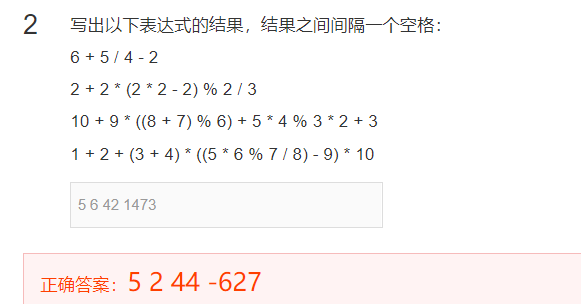# 求助！求解求解求解求解...全文
44 4 打赏 收藏 举报

4 条回复

forever74 08-17

• 打赏
• 举报

2+2*(22-2)%2/3 = 2+2(4-2)%2/3 = 2+22%2/3 = 2+4%2/3 = 2+0/3 = 2
10+9
((8+7)%6)+54%32+3 = 10+93+20%32+3 = 10+27+22+3=37+4+3=44
1+2+(3+4)
((5*6%7/8)-9)10=3+7(30%7/8-9)10=3+7(-9)*10=3-630=-627

• 打赏
• 举报

Oorik 08-16

• 打赏
• 举报

Oorik 08-16

9=27 ;
54=20; 20%3=2 ; 22 =4 ;
27+10=37 ;4+3 =7
7+37=44

• 打赏
• 举报【安装包】Cplex求解代码 PSO求解多目标优化问题代码matlab_方程求解_COMSOL-求解器配置
COMSOL-求解器配置代码 离散型遗传算法求解组合优化代码